AIMAll
(Version 19.10.12)

1997-2019 (aim@tkgristmill.com)
AIMQBAIMQB is the "driver" application for setting up and running most QTAIM calculations with AIMAll.  AIMQB automatically runs, with various options, a full QTAIM numerical analysis of one or more molecular wavefunction using the programs AIMExt, AIMInt and AIMSum.  Results from these programs are summarized in easy-to-read .sum files (a .sum file is a text file with the same base name as the corresponding wavefunction file but with the extension .sum).  Results from these programs can also be interactively displayed using AIMStudio.  Standard output (such as calculation progress) from AIMQB calculation steps can be displayed in an AIMQB log window or (when running AIMQB from a command-line) in a console window (Windows) or terminal (Mac OS X and Linux).

AIMQB can be run either in Dialog mode (GUI mode) or in Command-Line mode (non-GUI mode).

The general usage statement for AIMQB is:  aimqb [options] [wfnfile | wfxfile | fchkfile]

AIMQB can be launched in Dialog mode from Windows, Mac OS X or Linux by double-clicking an AIMQB application iconor by dragging and dropping a wavefunction file onto an AIMQB application icon.

AIMQB can also be launched in Dialog mode from the "Run" menu of AIMStudio.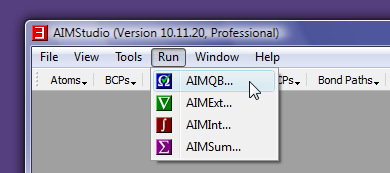AIMQB can be launched from a command-line either in Dialog mode or Command-Line mode.  A detailed description of using AIMQB with options from a command-line is given here.

## AIMQB Dialog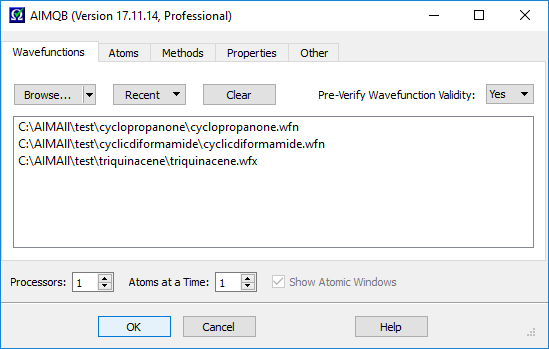Launching AIMQB by double-clicking an AIMQB application iconor from the "Run" menu of AIMStudio or by running aimqb from a command-line (without the -run or -nogui arguments) will display an AIMQB Dialog.  An AIMQB Dialog consists of five tabbed pages:

 Page Purpose Wavefunctions Choose the wavefunction files to use for calculations Atoms Specify the set of atoms to calculate Methods Specify the methods and method parameters to use for the calculations Properties Specify what properties to calculate Other Miscellaneous

The "Wavefunctions" page is shown above while the "Atoms", "Methods", "Properties" and "Other" pages are shown below.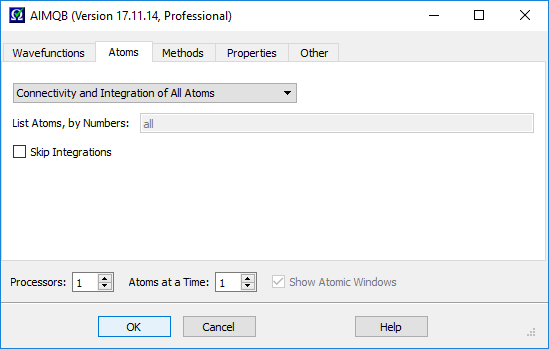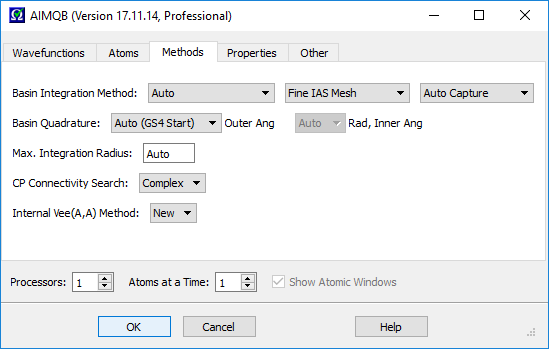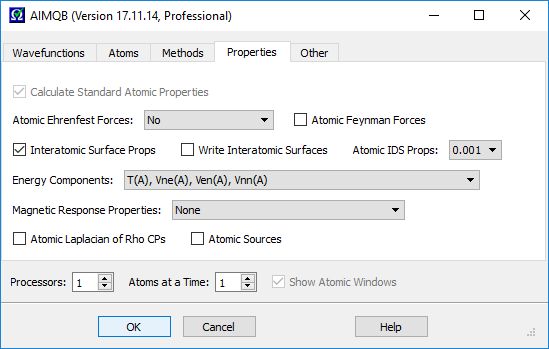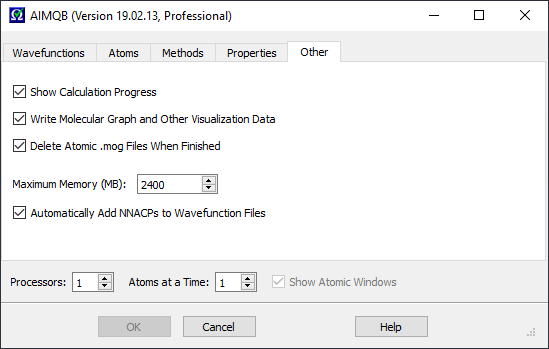#### AIMQB Dialog / Wavefunctions / List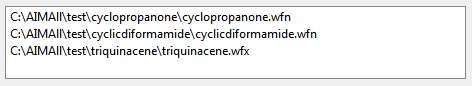The Wavefunction List contains the file paths of the wavefunctions (.wfn files, .wfx files, .fch files or .fchk files) on which AIMAll calculations are to be done.  The Wavefunction List must contain at least one wavefunction file path.  If more than one wavefunction file is in the Wavefunction List, the wavefunctions will be processed serially, i.e., in batch mode, beginning with the first wavefunction in the List and ending with the last Wavefunction in the List.  Wavefunctions can be added to the List using the "Browse" button or the "Recent" button.  Wavefunctions can also be added to the Wavefunction List by dragging the file icons from outside of AIMQB (e.g., from an Explorer window or desktop) and dropping them into the Wavefunction List.  Selected wavefunctions can be removed from the Wavefunction List using the Delete keyboard key.  The order of the Wavefunctions in the List can be rearranged by dragging and dropping files within the list.  The Wavefunction List can be cleared using the "Clear" button.  Note that the AIMQB Dialog settings will be used for each of the wavefunctions in the List.  If non-default settings are specified, care must be taken to ensure that they are appropriate for each of the wavefunctions in the Wavefunction List.

#### AIMQB Dialog / Wavefunctions / Browse...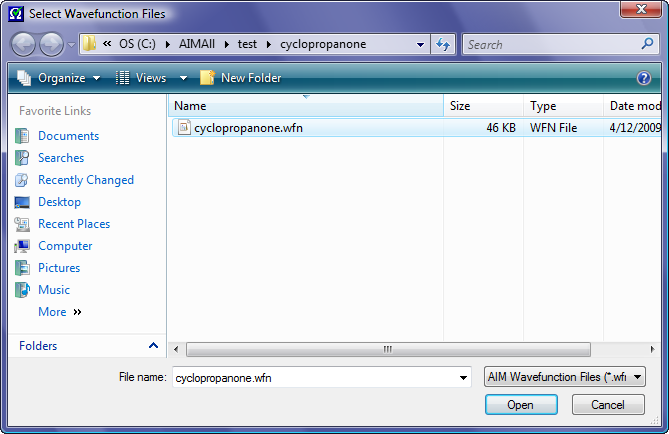Selecting the "Browse" button will launch a native Windows, Mac OS X or Linux file open dialog for selecting one or more wavefunction files in a directory to be added to the Wavefunction List to run the AIMAll calculations on.  To add wavefunction files from multiple directories to the Wavefunction List, just "Browse" multiple times.

#### AIMQB Dialog / Wavefunctions / External Drag-and-Drop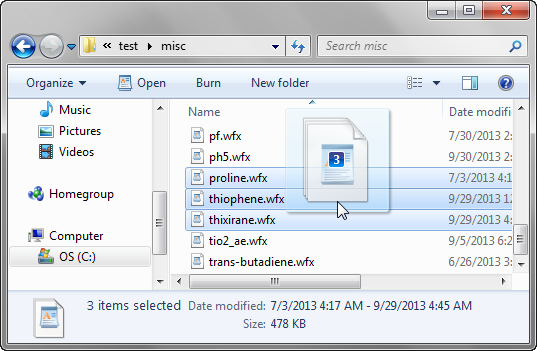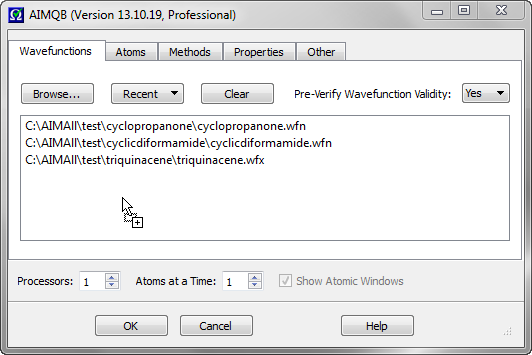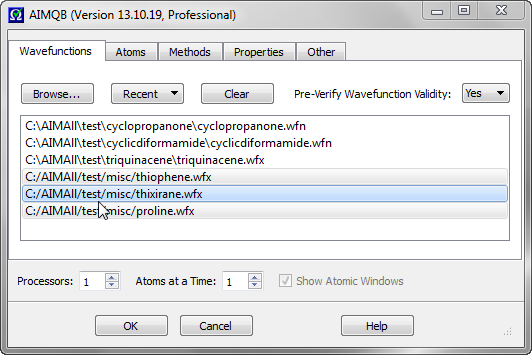Wavefunction can be added to the Wavefunction List by dragging the file icons from outside of AIMQB (e.g., from an Explorer window or desktop) and dropping them into the Wavefunction List.  In the above example, proline.wfx, thiophene.wfx and thixirane.wfx are added to the Wavefunction List by dragging their file icons from a Windows explorer window and dropping them into the List.

#### AIMQB Dialog / Wavefunctions / Internal Drag-and-Drop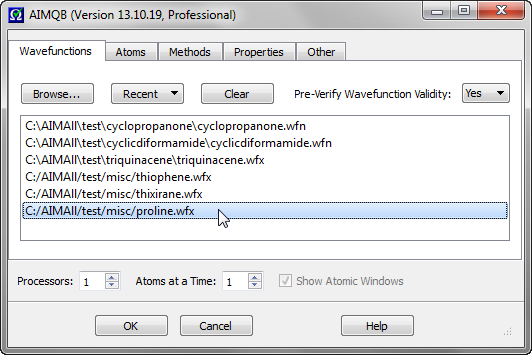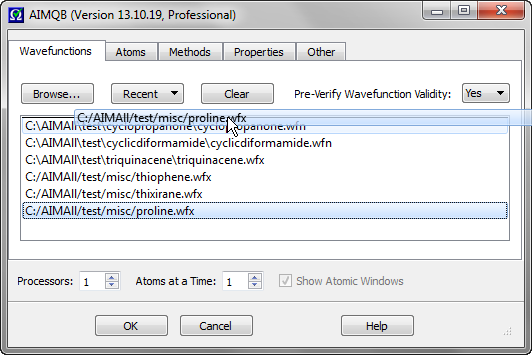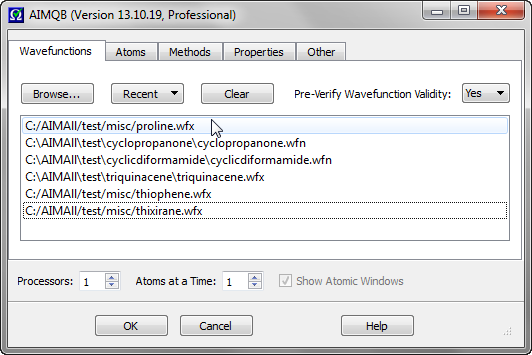The order of the Wavefunctions in the List can be rearranged by dragging and dropping files within the list.  In the above example, proline.wfx is moved from the bottom of the List to the top.

#### AIMQB Dialog / Wavefunctions / Recent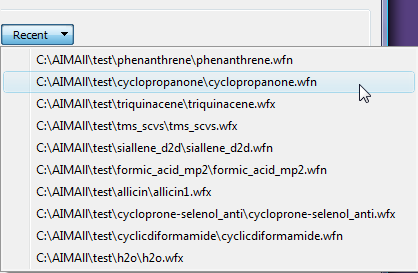The "Recent" menu presents the 10 most recent wavefunction files that were used with AIMQB.  Selecting a wavefunction file from the "Recent" menu will add it to the Wavefunction List.

#### AIMQB Dialog / Wavefunctions / Clear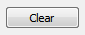Clicking the "Clear" button will clear the Wavefunction List.

#### AIMQB Dialog / Wavefunctions / Pre-Verify Wavefunction Validity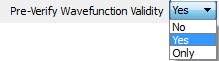For each AIM wavefunction (.wfn file or .wfx file) in the Wavefunction List, controls whether a verification of the validity of the AIM wavefunction will be done before proceeding with the AIMAll calculations for that wavefunction.  Most importantly, an orthonormality check of the MOs of the AIM wavefunction is done.  Default is "Yes", meaning the verification will be done and if the wavefunction is not acceptable then an error message will be issued and the AIMAll calculations will not proceed for that wavefunction.  Specifying "No" will skip the verification, which is NOT advisable.  Specifying "Only" will perform the verification of the wavefunction, inform the user of whether the wavefunction is acceptable or not and then stop, i.e., no calculations will be done even if the wavefunction is acceptable.

#### AIMQB Dialog / Wavefunctions / Files of type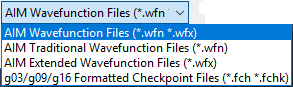AIMQB accepts three types of wavefunction data files.

• A traditional AIM wavefunction file (extension .wfn) that can be created by many popular ab initio quantum chemistry programs.
• An extended AIM wavefunction file (extension .wfx), which is a new wavefunction file format originally defined for AIMAll.  The content of a .wfx file is a superset of that in a .wfn file and it also eliminates some fixed formatting issues with traditional .wfn files.
• A g03 or g09 or g16 formatted checkpoint file (extension .fch or .fchk).  In this case, AIMQB will simply convert the .fch(k) file to a corresponding .wfx or .wfn file that will actually be used by the other programs of AIMAll.

#### AIMQB Dialog / Wavefunctions / FChk to AIM Wavefunction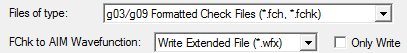When a g03 or g09 or g16 formatted checkpoint file is specified as a wavefunction file, the user has a choice of creating a corresponding .wfx or .wfn file that will be used by the other programs of AIMAll.  The default is to create a .wfx file.  When "Only Write" is checked, then AIMQB will terminate after the .wfx or .wfn file is created, instead of proceeding with the usual AIMAll calculations.

#### AIMQB Dialog / Atoms / List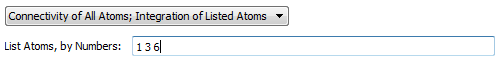These widgets control which atoms to determine critical point connectivity for and calculate atomic properties for.  The default is to determine connectivity and atomic properties of all atoms.  The option "Connectivity of All Atoms; Integration of Listed Atoms" will calculate a full molecular graph but will only calculate atomic properties of the listed atoms.  The option "Connectivity and Integration of Listed Atoms" (recommended for reruns of problem atoms following an all atom run) will only determine the critical point connectivity and atomic properties of the listed atoms, i.e., the full molecular graph will not be (re)calculated.  In the last case, if the full critical point connectivity and other critical point data is already present in a .mgp or .mgpviz file from a previous AIMQB run, then that data will be preserved if the critical points are validated.  When listing atoms to calculate, use a space-separated list of atom numbers.  For example, 1 3 6.

Note that when calculation of Vee(A,B) is requested for "Energy Components", then Vee(A,B) results will be calculated for all atomic pairs from the specified list of atoms.  For atoms that do not appear in the "Atoms to Calculate" list but whose .mog files are present from a previous calculatiom (because "Delete Atomic .mog Files When Finished" was not checked for example), then Vee(A,B) results involving these other atoms and all atoms in the "Atoms to Calculate" list will also be calculated.

#### AIMQB Dialog / Atoms / Skip Integrations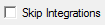This checkbox controls whether to skip the atomic integrations.  The default is unchecked (false).  Note that the atomic integration input files (.inp) for the AIMInt program will still be written even if this box is checked, as will a .bat script (Windows) or .sh script (Linux and Mac OS X) for running the atomic integrations.

#### AIMQB Dialog / Methods / Basin Integration Method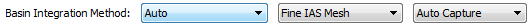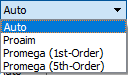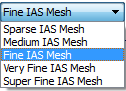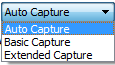Basin integration method, i.e., method to use to determine the surface of the atom (which is defined by its interatomic surfaces) and the intersections of the atomic integration rays with the atomic surface.  AIMAll supports four different atomic basin integration methods:  "Auto", "Proaim", "Promega (1st-order)" and "Promega (5th-order)".  The "Auto" method is the default, which means AIMAll will automatically decide which of the remaining methods to use to achieve atomic integrations results of good numerical accuracy.  The IAS Mesh options are applicable to the "Auto" and "Proaim" methods while the "Capture" options are applicable to the "Auto" and "Promega" methods.

NOTE: As of AIMAll (Version 12.05.09), the default atomic integration method and defaults for atomic integration parameters have been changed to "Auto", which means that AIMAll will automatically determine the appropriate initial atomic integration method and integration parameters for each atom and automatically perform appropriate recalculations of "Problem Atoms" as necessary to achieve atomic integration results of good numerical accuracy.  Sometimes, however, advanced users may wish to choose a specific integration method and / or specific integration parameters, in which case the following discussion is of interest.

For AIMAll, much time and effort has been spent on improving the robustness of the default "Proaim" Basin Integration method (compared to its original implementation in AIMPAC) since this method is the most efficient.   Development work on further improvement of the "Proaim" algorithm continues, with the goal of making it even more robust.

It is highly recommended that the default "Proaim" Basin Integration method and the default Basin Quadrature parameters always be tried first since they usually work quite well for most atoms and are relatively fast.

Only the "Proaim" method can be used to calculate interatomic surface properties.

In the event that integration results for one or more atoms are not sufficiently accurate (or are unavailable) using the default integration options, you can rerun AIMQB for just those problem atoms, using different integration options along with the "Atoms to Calculate" fields near the bottom of the AIMQB dialog.

If an atom has a "potentially significant" but not "significant" or serious integration error, simply increasing the "Outer Ang" Basin Quadrature while still using the "Proaim" Basin Integration method might help, and is not too expensive.

The "IAS Mesh" options are specific to the "Proaim" method and control the maximum allowed spacing between adjacent interatomic surface (IAS) paths and hence the fineness of the IAS triangulation and the accuracy of the intersections of the basin integration rays with the IASs.  The default "Fine IAS Mesh" is usually quite sufficient for accurate atomic integrations but the "Very Fine" or "Super Fine" IAS Meshes in conjunction with large quadratures may sometimes be useful for very accurate atomic integrations.

Using the "Promega (1st-Order)" basin integration method is usually a good choice for atoms whose integration results are significantly in error or unavailable due to a failure using the default "Proaim" method.  Note, however, that the "Promega (1st-Order)" method is significantly more expensive than the "Proaim" method.

The "Promega (5th-Order)" method is similar to the "Promega (1st-Order)" method except that up to 5 intersections of the integrations rays with the atomic surfaces are searched for.  While the "Promega (5th-Order)" is at least as accurate and sometimes more accurate than the "Promega (1st-Order)" method, it is also slower.

The "Extended Capture" option can be used to modestly speed up the "Promega (1st-Order)" method and significantly speed up the "Promega (5th-Order)" method, at the very slight risk of causing the methods to fail.  The default "Auto Capture" means the Extended Capture will be used for Promega (5th-Order) method but not for Promega (1st-Order).

Once a rerun of AIMQB for just the specified problem atoms is done, the sumfile will automatically be properly upated to include the results for the newly calculated atoms.

Note that for molecules with very diffuse charge distributions, such as some anions or excited states, increasing the maximum atomic integration radius may be necessary to obtain good accuracy, independent of the basin integration method and basin quadrature parameters.  This section assumes that the maximum atomic integration radius has been set sufficiently large.

#### AIMQB Dialog / Methods / Basin Quadrature

NOTE: As of AIMAll (Version 12.05.09), the default atomic integration method and defaults for atomic integration parameters have been changed to "Auto", which means that AIMAll will automatically determine the appropriate initial atomic integration method and integration parameters for each atom and automatically perform appropriate recalculations of "Problem Atoms" as necessary to achieve atomic integration results of good numerical accuracy.  Sometimes, however, advanced users may wish to choose a specific integration method and / or specific integration parameters, in which case the following discussion is of interest.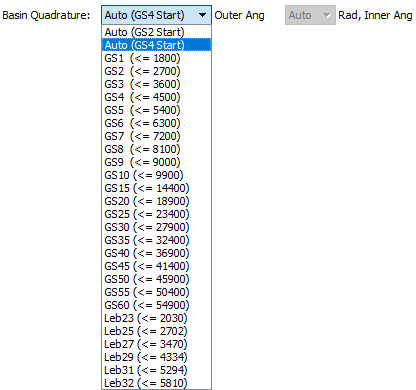Atomic basin properties are calculated using 3D numerical integration.  For the purposes of the 3D integration, the atom is broken up into two major regions:  an "Inner" region that is a sphere (Beta sphere) centered on the nucleus and contained within the atom; and an "Outer" region that is the rest of the atom.  The "Outer" region of an atom in a molecule is bounded by one or more interatomic surfaces and is generally complex in shape.  In AIMAll, both the "Inner" region and "Outer" region integrals are done using a combination of radial and angular numerical integration quadratures.

The most important Basin Quadrature control is the "Outer Ang" control, which determines the angular integration quadrature to be used for the "Outer" region of the atom.  The first two entries in the "Outer Ang" combobox ("Auto (GS2 Start)" and "Auto (GS4 Start)") automatically determine a sufficiently large GS angular quadrature based in part on the results obtained for L(A) ((-1/4) times the atomic integral of the Laplacian of the electron density, an integral which should be zero) using that angular quadrature.  The two "Auto" methods differ only in the starting GS grid to use, GS2 or GS4.  The next twenty entries in the "Outer Ang" combobox (GS1, GS2, ..., GS10, GS15, GS20, ..., GS60) correspond to specific GS angular quadratures of increasing size.  "GS2 (<= 2700)" means that up to 2700 GS angular grid points will be used per atom (depending on the type of atom and number of bonds).  The last six entries of the "Outer Ang" combobox (Leb25, Leb27, Leb29, Leb30, Leb31, Leb32) correspond to specific Lebedev quadratures of increasing size for the "Outer Ang" integration.  "Leb25 (<= 2702)" means that up to 2702 Lebedev angular grid points will be used per atom (depending on the type of atom and number of bonds).  The default "Outer Ang" choice is "Auto (GS4 Start)", meaning that the AIMAll will start with a GS4 grid and only increase it if necessary.  The larger grids may be useful for more accurately integrating atoms with very complex (but still accurately determinable) surface shapes.  But larger integration grids are also more expensive, especially for calculations involving Vee(A), Vee(A,A) and Vee(A,B).  For most users and use cases, it is strongly advisable to stick with one of the "Auto" methods.

As of AIMAll (Version 14.06.21), the "Rad, Inner Ang" Basin Quadrature control has been disabled, with the "Auto" setting always being used.

Note that for molecules with very diffuse charge distributions, such as some anions or excited states, increasing the maximum atomic integration radius may be necessary to obtain good accuracy, independent of the basin integration method and basin quadrature parameters.  This section assumes that the maximum atomic integration radius has been set sufficiently large.

#### AIMQB Dialog / Methods / Max. Integration Radius

NOTE: As of AIMAll (Version 12.05.09), the default atomic integration method and defaults for atomic integration parameters have been changed to "Auto", which means that AIMAll will automatically determine the appropriate initial atomic integration method and integration parameters for each atom and automatically perform appropriate recalculations of "Problem Atoms" as necessary to achieve atomic integration results of good numerical accuracy.  Sometimes, however, advanced users may wish to choose a specific integration method and / or specific integration parameters, in which case the following discussion is of interest.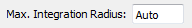The maximum atomic integration radius editor control determines the maximum radial boundary, in atomic units, that can be used for the radial integrations of the Outer region of an atom.  The default is 13.0.  Larger values may sometimes be necessary for some anions or excited states.  Any number between 7.0 and 30.0 is allowed.

#### AIMQB Dialog / Methods / CP Connectivity Search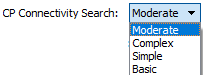This combobox controls the intensity of the search for connectivity between the electron density critical points (CPs) found, especially connectivity between bond critical points (BCPs) and ring critical points (RCPs).  "Moderate" is the default and searches for all CP connectivities using a thorough procedure that is almost always sufficient but can be expensive for systems with many atoms and many RCPs.  "Complex" uses a more thorough but more expensive algorithm than "Moderate".  "Simple" searches for all CP connectivities using a somewhat less thorough and less expensive procedure than that corresponding to "Moderate" and "Complex".  "Basic" searches only for BCP connections to nuclear attractors critical points (NACPs) and non-nuclear attractor critical points (NNACPs), i.e., bond paths.  "Basic" is relatively fast in all cases but may make the "Proaim" basin integration method slower, with the very slight possibility of reduced accuracy or failure (in which case one of the "Promega" methods will be used if "Auto" was chosen for the "Basin Integration Method").

#### AIMQB Dialog / Methods / Internal Vee(A,A) Method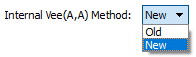Determines whether to use AIMAll's new algorithm for Vee(A,A) calculations, or AIMAll's old algorithm.  Default is "New", which is faster and typically more accurate than the old algorithm.

#### AIMQB Dialog / Properties / Atomic Ehrenfest Forces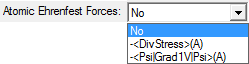This combobox controls whether and how to calculate atomic Ehrenfest forces.  The default is not to calculate any atomic Ehrenfest forces.  -<DivStress>(A) means the atomic Ehrenfest forces will be calculated in terms of minus the divergence of the stress tensor.  -<Psi|Grad1V|Psi>(A) means the atomic Ehrenfest forces will be calculated in terms of the one-electron gradient of the potential energy operator, which is very expensive (more expensive than Vee(A) calculations).  -<DivStress>(A) will also be calculated when -<Psi|Grad1V|Psi>(A) is selected.

#### AIMQB Dialog / Properties / Atomic Feynman Forces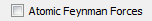This checkbox controls whether to print atomic contributions to the Feynman electrostatic forces on each of the nuclei in the molecule to the .sum and .sumviz files.  The default is unchecked (false).  Atomic Feynman force data can be quite large (O(N**2)) and users uninterested in this data should consider leaving this box unchecked to avoid cluttering the .int, .sum and .sumviz files.

#### AIMQB Dialog / Properties / Interatomic Surface Props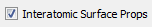This checkbox controls whether to calculate interatomic surface (IAS) properties such as IAS areas (out to the 0.0004, 0.001 and 0.002 isodensity surfaces), the number of electrons per unit length, the kinetic energy per unit length, etc.  The default is checked (true).  This option can only be used in conjunction with the default "Proaim" atomic basin integration method.  To calculate the "Surface Virial" energy and the surface "pressure" (surface integral of the Ehrenfest Force density) for each of the IASs, check this box and also select the -<DivStress>(A) option or the -<Psi|Grad1V|Psi>(A) option for "Atomic Ehrenfest Forces".

#### AIMQB Dialog / Properties / Write Interatomic Surfaces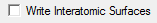This checkbox controls whether to write interatomic surface (IAS) data (both GradRho paths and IAS / integration ray intersection data) to atomic .iasviz files, primarily for use with the AIMStudio visualization program.  Default is unchecked (false).  For a wavefunction file named abc.wfx, the .iasviz files will be written to the abc_atomicfiles subdirectory of the directory containing abc.wfx.  These .iasviz files can be quite large (several MB per IAS) and, for large molecules, calculations using this option should typically only be done for a subset of the atoms in the molecule.

Note:  Multiprocessor calculation features and all visualization-related features, such as writing .iasviz files and displaying their data in AIMStudio, require an AIMAll Professional license for wavefunctions with more than 12 atoms or more than 400 primitive basis functions.

#### AIMQB Dialog / Properties / Atomic IDS Props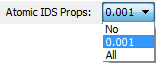This combobox controls the calculation of atomic isodensity surface (IDS) properties - particularly electrostatic potential (ESP) properties, which can be expensive.  The default is "0.001", which means calculate ESP properties and other properties on the atomic portions of the molecule's 0.001 a.u. isodensity surface.  Selecting "No" means that ESP properties will not be calculated on any isodensity surfaces.  Selecting "All" means calculate ESP properties and other properties on the atomic portions of the molecule's 0.0004, 0.001 and 0.002 a.u. isodensity surfaces.  Note that the atomic isodensity surface areas are always calculated.

#### AIMQB Dialog / Properties / Energy Components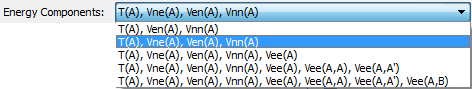This combobox controls which atomic energy components to calculate.  Note that A represents the current atom being integrated, B represents another atom in the molecule and A' represents the sum of all other atoms in the molecule.  The energy components are as follows:

• T(A) is the electron kinetic energy of atom A.
• Ven(A) = Ven(A,Mol) is the attraction energy between the electron density distribution of atom A and all of the nuclear charges in the molecule.
• Ven(A,A) (also called VenO(A)) is the attraction energy between the electron density distribution of atom A and the nucleus of atom A.
• Ven(A,A') is the attraction energy between the electron density distribution of atom A and the nuclei of all other atoms in the molecule.  Ven(A,A') = Ven(A) - Ven(A,A).
• Vnn(A,A') is the repulsion energy between the nucleus of atom A and the other nuclei of the molecule
• Vnn(A) is the contribution of atom A to the nuclear-nuclear repulsion energy of the molecule.  The definition of Vnn(A) differs between the "Virial-Based" total atomic energy approach and the IQA total atomic energy approach.  In the former case Vnn(A) is calculated from all other energy components assuming the satisfaction of the full atomic virial theorem.  For IQA, Vnn(A) = Vnn(A,A')/2 is simply one-half of the repulsion energy between the nucleus of atom A and the other nuclei of the molecule.
• Vne(A) = Vne(A,Mol) is the attraction energy between the nucleus of atom A and the electron density distribution of the entire molecule.  This quantity only appears in the IQA atomic energy expression.
• Vne(A,A) = Ven(A,A) is the attraction energy between the nucleus of atom A and the electron density distribution of atom A.
• Vne(A,A') is the attraction energy between the nucleus of atom A and the electron density distribution of all other atoms in the molecule.  Vne(A,A') = Vne(A) - Vne(A,A).
• Vee(A) = VeeC(A) + VeeX(A) = Vee(A,A) + Vee(A,A')/2 = VeeC(A,A) + VeeX(A,A) + VeeC(A,A') + VeeX(A,A') is the two-electron interaction energy of Atom A with itself plus half of the two-electron interaction energy between atom A and other atoms of molecule.  Calculations of Vee(A) can be time-consuming.
• VeeC(A) = VeeC(A,Mol) is the Coulomb part of Vee(A).
• VeeX(A) = VeeX(A,Mol) is the "exchange-correlation" part of Vee(A).  The formula for VeeX depends on the underlying model of the wavefunction being used.  For Hartree-Fock and post Hartree-Fock wavefunctions, VeeX represents the usual two-electron non-local/Hartree-Fock-like exchange energy formula.  For the DFT model B3LYP, VeeX represents the B3LYP exchange-correlation functional.  For the DFT model LSDA, VeeX represents the LSDA exchange-correlation functional.  For the DFT model M062X, VeeX represents the M062X exchange-correlation functional.  For the DFT model PBE, VeeX represents the PBE exchange-correlation functional.  For the DFT model PBE0, VeeX represents the PBE0 exchange-correlation functional.
• Vee(A,A) = VeeC(A,A) + VeeX(A,A) = is the two-electron interaction energy of atom A with itself.  Calculations of Vee(A,A) can be very time-consuming.
• Vee(A,A') = VeeC(A,A') + VeeX(A,A') is the two-electron interaction energy between atom A and all other atoms in the molecule.  Vee(A,A') = Vee(A) - Vee(A,A).
• Vee(A,B) = VeeC(A,B) + VeeX(A,B) is the two-electron interaction energy between atoms A and B.  Calculations of Vee(A,B) can be time-consuming and are always accompanied by calculations of the other diatomic interaction energy contributions Ven(A,B), Vne(A,B) and Vnn(A,B).

AIMAll supports two QTAIM total atomic energy definitions:  The traditional "Virial-Based" total atomic energy and the newer "Interacting Quantum Atoms (IQA)" total atomic energy based on the work of Pendas et al.

The "Virial-Based" total atomic energy of atom A is defined (by analogy with the molecular total energy expression from the molecular virial theorem) as:

E(A) = T(A) + Ven(A) + Vee(A) + Vnn(A) = -T(A) + W(A)

where Vnn(A) is defined by the QTAIM atomic virial theorem (2T(A) + Ven(A) + Vee(A) + Vnn(A) - W(A) = 0) as:

Vnn(A) = -2T(A) - Ven(A) - Vee(A) + W(A)

and where W(A) is the contribution of atom A to the nuclear virial of the energy-gradient-based forces on the nuclei.  W(A) is a heuristically defined "atomic response property" that is assumed to vanish for equilibrium and other stationary point molecular geometries, just as W(Mol) does.

"Virial-Based" total atomic energies E(A) defined as -T(A) + W(A) are totally determined by the 1-electron density matrix (1EDM) and are relatively easy to calculate, but they are beset with the practical problem that typical approximate wavefunctions do not satisfy the molecular virial theorem (nor the more difficult to satisfy atomic virial theorems) and in such cases "Virial-Based" total atomic energies E(A) defined as -T(A) + W(A) will not be additive to give the total molecular energy E.

The IQA total atomic energy of atom A, E_IQA(A), is defined as:

E_IQA(A) = T(A) + (1/2)Ven(A,Mol) + (1/2)Vne(A,Mol) + Vee(A) + (1/2)Vnn(A,A')

= T(A) + Ven(A,A) + Vee(A,A) + (1/2)Ven(A,A') + (1/2)Vne(A,A') + (1/2)Vee(A,A') + (1/2)Vnn(A,A')

IQA total atomic energies are relatively costly to calculate (because of Vee(A)) but are, in principle, well-defined for any wavefunction and geometry and the potential energy terms can all be expressed in terms of individual interactions of atom A with itself and with each of the other atoms B in the molecule, which might be useful for interpretation:

Ven(A,A') = Sum_Over_B_Not_A[Ven(A,B)]

Vne(A,A') = Sum_Over_B_Not_A[Vne(A,B)]

Vnn(A,A') = Sum_Over_B_Not_A[Vnn(A,B)]

Vee(A,A') = VeeC(A,A') + VeeX(A,A') = Sum_Over_B_Not_A[Vee(A,B)]

VeeC(A,A') = Sum_Over_B_Not_A[VeeC(A,B)]

VeeX(A,A') = Sum_Over_B_Not_A[VeeX(A,B)]

To calculate IQA total atomic energies, it is sufficient to use the third option ("T(A), Vne(A), Ven(A), Vnn(A), Vee(A)"), which is not too expensive.  To calculate individual interatomic interaction energy contributions it is necessary to use the fifth option ("T(A), Vne(A), Ven(A), Vnn(A), Vee(A), Vee(A,A), Vee(A,A'), Vee(A,B)"), which can be quite expensive.

For post-Hartree-Fock, natural orbital "wavefunctions" (which are presently all that is typically available in practice for post Hartree-Fock methods from most program) the Muller approximation of the two-electron density matrix (2EDM) in terms of natural orbitals of the one-electron density matrix (1EDM) is used to calculate 2EDM-dependent properties (i.e., Vee contributions and electronic localization and delocalization properties). For HF single-determinant wavefunctions the expression used for the 2EDM is exact. What this means is that for such post-Hartree-Fock wavefunctions, the IQA total atomic energies will not be additive to the give the molecular energy because of the approximation of the 2EDM.

VeeX represents the exchange-correlation functional for the wavefunction's underlying model.  For Hartree-Fock wavefunctions, VeeX is the two-electron Hartree-Fock exchange functional.  For wavefunctions of supported DFT models (currently LSDA and B3LYP and M062X and PBE and PBE0), VeeX is the actual exchange-correlation functional of the corresponding DFT model and atomic contributions VeeX(A) to VeeX are explicitly calculated and unambiguous.  However, since VeeX is at least partly just a one-electron functional for DFT models, the partitioning of VeeX(A) into interatomic (VeeX(A,B) and VeeX(A,A')) and intraatomic (VeeX(A,A)) contributions is ambiguous.  Currently, interatomic contributions VeeX(A,B) and VeeX(A,A') are calculated using the Hartree-Fock exchange functional while the intratomic contribution VeeX(A,A) is calculated as VeeX(A) - VeeX(A,A').  For wavefunctions of non-supported DFT models (currently models other than LSDA or B3LYP or M062X or PBE or PBE0), VeeX is (incorrectly) assumed to be the Hartree-Fock exchange functional and the atomic energies E_IQA(A) will not be correct and will not sum to the correct molecular energy.

It is fair to say that use of QTAIM atomic energies and some of their components should be done only with great care and with a full understanding of what they are and what they are not.  In practice (i.e., with the wavefunctions that are actually routinely available), the only truly well-defined, additive QTAIM total atomic energies are IQA total atomic energies calculated from Hartree-Fock and supported KS-DFT (currently LSDA or B3LYP or M062X or PBE or PBE0) single-determinant wavefunctions at any geometry and virial-based total atomic energies with any Hartree-Fock or post-Hartree-Fock wavefunction (meaning not KS-DFT wavefunctions) that satisfies the molecular virial theorem and that preferably is for an equilibrium geometry or other stationary point geometry (to avoid the necessity of heuristically approximating W(A)).

The default "Energy Components" option is "T(A), Ven(A), Vne(A) and Vnn(A)".

A consequence of using the default energy component option "T(A), Ven(A), Vne(A) and Vnn(A)" or any higher Energy Component option that involves Vne(A) is that various electrostatic potential (ESP) properties - such as minimum ESP, maximum ESP and surface-integrated ESP - of the 0.001 (and optionally 0.0004 and 0.002) atomic isodensity surfaces can be calculated.

#### AIMQB Dialog / Properties / Magnetic Response Properties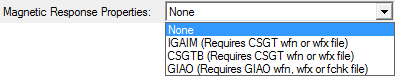This combobox controls which method, if any, to use to calculate atomic magnetic response properties such as atomic net currents, atomic contributions to NMR shielding tensors and atomic contributions to magnetizabilities.  The default is not to calculate atomic magnetic response properties.  The CSGTB and IGAIM methods requires a CSGT-based magnetic wavefunction file.  The GIAO method requires a GIAO-based magnetic wavefunction file or a .fch(k) file with GIAO based density matrix data.

#### AIMQB Dialog / Properties / Atomic Laplacian of Rho CPs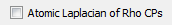This checkbox controls whether to locate and characterize critical points of the Laplacian of Rho (DelSqRho), where Rho is the electron density.  The default is unchecked (false).  The Laplacian of Rho critical point data is determined an atom at a time and the data is written to atomic result visualization files ending with the extension .agpviz.  For a wavefunction file named abc.wfx, the .agpviz files will be written to the abc_atomicfiles subdirectory of the directory containing abc.wfx.  These .agpviz files can be opened in AIMStudio in order to visualize Laplacian of Rho critical points (and corresponding atomic graph gradient paths) and their properties in 3D windows and in interactive Tables.

Note that calculation of Laplacian of Rho critical point data typically significantly increases atomic computation times and users uninterested in this data should avoid checking this box.

Note that in AIMAll, topological analyses of the actual Laplacian of Rho (DelSqRho) are reported (as opposed to the more traditional reporting of minus the Laplacian of Rho (-DelSqRho) analyses).  This is done to be consistent with the use of the Laplacian of Rho in other areas of AIMAll (plotting, for example).

The signs of the Hessian of DelSqRho and its eigenvalues are opposite to the signs of the Hessian of -DelSqRho and its eigenvalues.  The signatures of critical points of DelSqRho are thus opposite in sign to those of -DelSqRho.

A (3,+3) critical point of DelSqRho (which is equivalent to a (3,-3) critical point of -DelSqRho) is a local minimum of DelSqRho and is a point of locally maximal "Charge Concentration" when DelSqRho is negative or a point of locally minimal "Charge Depletion" when DelSqRho is positive.

A (3,-3) critical point of DelSqRho (which is equivalent to a (3,+3) critical point of -DelSqRho) is a local maximum of DelSqRho and is a point of locally maximal "Charge Depletion" when DelSqRho is positive or a point of locally minimal "Charge Concentration" when DelSqRho is negative.

A (3,+1) critical point of DelSqRho is a (3,-1) critical point of -DelSqRho.

A (3,-1) critical point of DelSqRho is a (3,+1) critical point of -DelSqRho.

Note:  Multiprocessor calculation features and all visualization-related features, such as writing .agpviz files and displaying their data in AIMStudio, require an AIMAll Professional license for wavefunctions with more than 12 atoms or more than 400 primitive basis functions.

#### AIMQB Dialog / Properties / Atomic Sources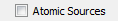This checkbox controls whether to calculate and print atomic source contributions to the electron density at electron density critical points and nuclei.  The default is unchecked (false).  Atomic source data can be quite large (O(N**2)) and users uninterested in this data should consider leaving this box unchecked to avoid cluttering the .int, .sum and .sumviz files.

#### AIMQB Dialog / Other / Show Calculation Progress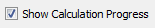This checkbox controls whether or not to show detailed calculation progress (especially atomic integration progress) in the AIMQB log window.  Default is checked.

#### AIMQB Dialog / Other / Write Molecular Graph and Other Visualization Data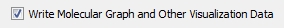This checkbox controls whether to write molecular graph and other visualization data to a .mgpviz file (which also contains the same critical point data as the *.mgp file) and a .sumviz file for use with the AIMStudio visualization program.  Default is checked.

Note:  Multiprocessor calculation features and all visualization-related features, such as writing and displaying molecular graphs, require an AIMAll Professional license for wavefunctions with more than 12 atoms or more than 400 primitive basis functions.

#### AIMQB Dialog / Other / Delete Atomic .mog Files When Finished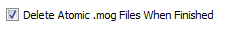This checkbox controls whether to delete the atomic .mog files used for Vee(A,A) and Vee(A,B) calculations when AIMQB is finished with the corresponding wavefunction.  Default is checked (true).  In some cases, users may wish to save the atomic .mog files to run additional Vee(A,B) calculations later, in which case this box should be unchecked.

#### AIMQB Dialog / Other / Maximum Memory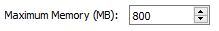Maximum memory (in megabytes) that can be used for potentially memory-intensive job steps, such as semi-analytic Vee(A) calculations and Vee(A,A) calculations on big wavefunctions.  For default job types, this value is not relevant.  Minimum is 800 MB.  For relevant job types and big wavefunctions, calculation times may decrease by increasing this maximum memory value.  Default is 800MB for 32-bit versions of AIMAll and 2400MB for 64-bit versions of AIMAll.

#### AIMQB Dialog / Processors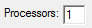The number of processors (shared memory only) to use for AIMExt and AIMInt jobs.  Default is 1.  Must be less than or equal to the number of logical processors of the system.  If more than one atom at a time is calculated, then the number of processors to use per atom will be (number of processors)/(number of atoms at a time).  Note that the most effective use of multiple processors for small wavefunctions is to calculate more than one atom at a time (e.g., Processors = 3 and Atoms at a Time = 3).  For large wavefunctions, using more than one processor per atomic calculation becomes effective (e.g., Processors = 3 and Atoms at a Time = 1).

Note:  Multiprocessor calculation features and all visualization-related features, such as writing and displaying molecular graphs, require an AIMAll Professional license for wavefunctions with more than 12 atoms or more than 400 primitive basis functions.

#### AIMQB Dialog / Atoms at a Time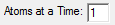The number of atoms to calculate at a time.  Must be less than or equal to the number of processors to use.  If the number of atoms to calculate at a time is less than the number of processors to use, then the number of processors to use per atom will be (number of processors)/(number of atoms at a time).  For example, if 4 processors and 2 atoms at a time are specified (and you have at least a 4 processor system), then two AIMInt jobs will be run at a time, each using 2 processors.  Note that the most effective use of multiple processors for small wavefunctions is to calculate more than one atom at a time (e.g., Processors = 3 and Atoms at a Time = 3).  For large wavefunctions, using more than one processor per atomic calculation becomes effective (e.g., Processors = 3 and Atoms at a Time = 1).

Note:  Multiprocessor calculation features and all visualization-related features, such as writing and displaying molecular graphs, require an AIMAll Professional license for wavefunctions with more than 12 atoms or more than 400 primitive basis functions.

#### AIMQB Dialog / Show Atomic Windows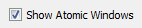When more than one atom is calculated at a time the main AIMQB log window, console window or terminal will only display a summary of the progress of the atomic integrations.  The "Show Atomic Windows" checkbox controls whether separate log windows should be shown for each atomic integration calculation.  The default is checked.  Note that atomic log windows will not be shown if -console or -nogui is specified.

Note:  Multiprocessor calculation features and all visualization-related features, such as writing and displaying molecular graphs, require an AIMAll Professional license for wavefunctions with more than 12 atoms or more than 400 primitive basis functions.

#### AIMQB Dialog / OK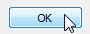Selecting the "OK" button will close the AIMQB dialog and start the AIMAll calculations on the wavefunction files in the Wavefunction List, the calculation progress being shown in an AIMQB log window by default.  If the Wavefunction List contains more than one wavefunction, the wavefunctions will processed serially, i.e., one at a time.

## AIMQB Log Window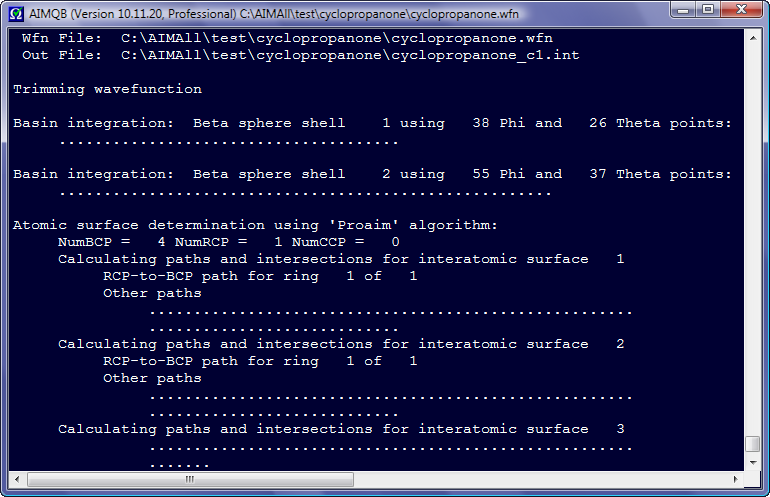After an AIMQB job is started, an AIMQB log window is shown by default and the progress of the AIMAll calculations for each wavefunction in the Wavefunction List is continuously written to this log window.  If you close an AIMQB log window while the AIMQB job is still running, you will be given the option of killing the AIMQB job or leaving it running without the log window.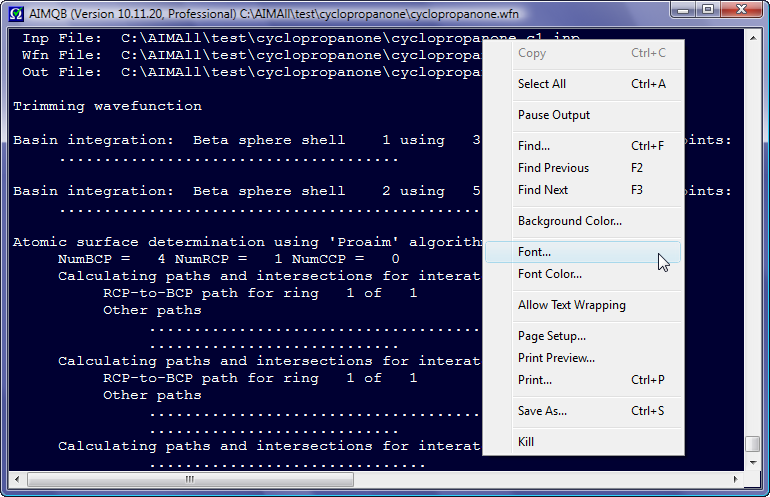Right-clicking in an AIMQB log window displays a context menu with options for controlling the appearance of the log window and other self-explanatory items.

## AIMQB Command-Line

AIMQB can be setup and / or run with any options entirely from a command-line interface.

For example, to run AIMQB using the default calculation setup options and the wavefunction file oxirane.wfn (assumed to be in the current directory), enter the following at a command prompt:

Windows:  c:\aimall\aimqb -nogui oxirane.wfn

Mac OS X:  /AIMAll/AIMQB.app/Contents/MacOS/aimqb -nogui oxirane.wfn

Linux:  /usr/local/AIMAll/aimqb.ish -nogui oxirane.wfn

The -nogui argument (equivalent to -console -run -warn=false) requests that no GUI actions occur, meaning that the AIMQB calculation setup dialog is bypassed and that the standard output (such as calculation progress) and any warning or error messages will be sent to the current console window or terminal instead of an AIMQB log window or warning or error message boxes.

Most of the option controls in the AIMQB dialog have corresponding command-line arguments.  To get a list of command-line arguments, enter the following at a command prompt:

Windows:  c:\aimall\aimqb -nogui -help

Mac OS X:  /AIMAll/AIMQB.app/Contents/MacOS/aimqb -nogui -help

Linux:  /usr/local/AIMAll/aimqb.ish -nogui -help

This will produce the following usage statement:
```AIMQB (Version 19.10.12, Professional)
Copyright (c) 1997-2019 by Todd A. Keith
AIMQB is a component of the AIMAll package ( http://aim.tkgristmill.com )

Command-line Usage:  aimqb [options] [wfxfile | wfnfile | fchkfile]

options:
[-bim=auto/proaim/promega/promega1/promega5]              (default is -bim=auto)
[-iasmesh=sparse/medium/fine/veryfine/superfine]          (default is -iasmesh=fine)
[-capture=auto/basic/extended]                            (default is -capture=auto)
[-boaq=auto/auto_gs2/auto_gs4/
gs1/gs2/gs3/gs4/gs5/gs6/gs7/gs8/gs9/gs10/gs15/
gs20/gs25/gs30/gs35/gs40/gs45/gs50/gs55/gs60/
leb23/leb25/leb27/leb29/leb31/leb32]               (default is -boaq=auto)
[-ehren=0/1/2]                                            (default is -ehren=0)
[-feynman=false/true]                                     (default is -feynman=false)
[-iasprops=false/true]                                    (default is -iasprops=true)
[-magprops=none/igaim/csgtb/giao]                         (default is -magprops=none)
[-source=false/true]                                      (default is -source=false)
[-iaswrite=false/true]                                    (default is -iaswrite=false)
[-atidsprops=no/0.001/all]                                (default is -atidsprops=0.001)
[-encomp=0/1/2/3/4]                                       (default is -encomp=1)
[-warn=false/true]                                        (default is -warn=true)
[-scp=false/true/some]                                    (default is -scp=some)
[-delmog=false/true]                                      (default is -delmog=true)
[-skipint=false/true]                                     (default is -skipint=false)
[-f2w=wfx/wfn]                                            (default is -f2w=wfx)
[-f2wonly=false/true]                                     (default is -f2wonly=false)
[-atoms=all] [-atoms=all_...] [-atoms=...]                (default is -atoms=all)
[-mir=auto/10.0/12.0/13.5/etc.]                           (default is -mir=auto)
[-cpconn=moderate/complex/simple/basic]                   (default is -cpconn=moderate)
[-intveeaa=old/new]                                       (default is -intveeaa=new)
[-atlaprhocps=false/true]                                 (default is -atlaprhocps=false)
[-wsp=false/true]                                         (default is -wsp=true)
[-nproc=1/2/3/etc.]                                       (default is -nproc=1)
[-naat=1/2/3/etc.]                                        (default is -naat=1)
[-shm_lmax=-1/0/1/2/etc.]                                 (default is -shm_lmax=5)
[-maxmem=800/1200/1800/2400/etc.]                         (default is -maxmem=800 for 32-bit)
(default is -maxmem=2400 for 64-bit)
[-verifyw=yes/no/only]                                    (default is -verifyw=yes)
[-saw=false/true]                                         (default is -saw=false)
[-autonnacps=false/true]                                  (default is -autonnacps=true)
[-help]
[-console]
[-newconsole]
[-run]
[-nogui]
```

The options have the following meaning:

-bim=auto/proaim/promega/promega1/promega5
Basin integration method, i.e., method to use to determine the surface of the atom (which is defined by its interatomic surfaces) and the intersections of the atomic integration rays with the atomic surface.  Default is auto, which means AIMAll will automatically decide which method to use to achieve atomic integrations results of good numerical accuracy.

-iasmesh=sparse/medium/fine/veryfine/superfine
This option controls the target spacing between adjacent IAS paths (the maximum allowable spacing is 10 percent larger than the target spacing) when the "Auto" or "Proaim" basin integration method is used.  The default IAS Mesh is "Fine", which is usually quite sufficient.  In conjunction with large Basin Quadratures, "Very Fine" or "Super Fine" IAS Meshes may sometimes be useful for very accurate atomic integrations.  Naturally, calculation time increases with the fineness of the IAS Mesh.

-capture=auto/basic/extended
Gradient path capture method (applicable to Auto and Promega basin integration methods).  Legal values are currently auto, basic and extended.  The default is "auto", which means extended capture will be used for "Promega (5th-Order) while basic capture will be used for "Promega (1st-Order)".   "Extended Capture" is significantly faster than "Basic Capture" for Promega(5th-Order) but has a very slight chance of failure.

-boaq=auto/auto_gs2/auto_gs4/gs1/gs2/gs3/gs4/gs5/gs6/gs7/gs8/gs9/gs10/gs15/gs20/gs25/gs30/gs35/gs40/gs45/gs50/gs55/gs60/leb23/leb25/leb27/leb29/leb31/leb32
Basin outer angular quadrature.  Default is auto, which means AIMAll will automatically decide which outer angular quadrature to use to achieve atomic integration results of good numerical accuracy.

-ehren=0/1/2
Whether and how to calculate atomic Ehrenfest forces.  Legal values are 0, 1 and 2.  The default is 0, meaning don't calculate any atomic Ehrenfest forces.  1 means yes, using the -<DivStress>(A) expression.  2 means yes, using the expensive -<Psi|Grad1V|Psi>(A) expression (as well as -<DivStress>(A)).

-feynman=false/true
Whether to print Feynman force data to the sum file.  Default is false.

-iasprops=false/true
This option controls whether to calculate interatomic surface (IAS) properties such as IAS areas (out to the 0.0004, 0.001 and 0.002 isodensity surfaces), the number of electrons per unit length, the kinetic energy per unit length, etc.  The default is true.  This option can only be used in conjunction with the default "Proaim" atomic basin integration method.  To calculate the "Surface Virial" energy and the surface "pressure" (surface integral of the Ehrenfest Force density) for each of the IASs, specify -iasprops=true and -ehren=1 or -ehren=2.

-magprops=none/igaim/csgtb/giao
Method to use to calculate atomic magnetic response properties.  Default is none.  These methods require magnetic wavefunction or fchk files.

-source=false/true
Whether to calculate and print atomic source contributions to the electron density at electron density critical points and nuclei.  The default is false.

-iaswrite=false/true
Whether to write interatomic surface data (paths and intersection data) to *.iasviz files for use with the AIMStudio visualization program.  Default is false.  For a wavefunction file named abc.wfx, the .iasviz files will be written to the abc_atomicfiles subdirectory of the directory containing abc.wfx.

Note:  Multiprocessor calculation features and all visualization-related features, such as writing .iasviz files and displaying their data in AIMStudio, require an AIMAll Professional license for wavefunctions with more than 12 atoms or more than 400 primitive basis functions.

-atidsprops=no/0.001/all
Controls the calculation of atomic isodensity surface (IDS) properties - particularly electrostatic potential (ESP) properties, which can be expensive.  The default is -atidsprops=0.001, which means calculate ESP properties and other properties on the atomic portions of the molecule's 0.001 a.u. isodensity surface.  Specifying -atidsprops=no means that ESP properties will not be calculated on any isodensity surfaces.  Specifying -atidsprops=all means calculate ESP properties and other properties on the atomic portions of the molecule's 0.0004, 0.001 and 0.002 a.u. isodensity surfaces.  Note that the atomic isodensity surface areas are always calculated.

-encomp=0/1/2/3/4
Atomic energy components to calculate.  Default is 1, i.e., calculate T(A), Vne(A), Ven(A) and Vnn(A).  0 means T(A), Ven(A), and Vnn(A).  2 means T(A), Vne(A), Ven(A), Vnn(A) and Vee(A).  3 means T(A), Vne(A), Ven(A), Vnn(A), Vee(A), Vee(A,A) and Vee(A,A').  4 means T(A), Vne(A), Ven(A), Vnn(A), Vee(A), Vee(A,A), Vee(A,A') and Vee(A,B).  A consequence of using the default energy component option of -encomp=1 or any higher Energy Component option that involves Vne(A) is that various electrostatic potential (ESP) properties - such as minimum ESP, maximum ESP and surface-integrated ESP - of the 0.001 (and optionally 0.0004 and 0.002) atomic isodensity surfaces can be calculated.

-warn=false/true
Show warning message boxes when appropriate.  The default is true.

-scp=false/true/some
Whether to show calculation progress in the log window, console window or terminal.  Default is true (show detailed calculation progress) in GUI mode and some (some calculation progress) in command-line mode.  Specifying -scp=false means that no calculation progress at all will be shown.

-delmog=false/true
Whether to delete the atomic .mog files used for Vee(A,A) and Vee(A,B) calculations when AIMQB is finished with the corresponding wavefunction.  Default is true.  In some cases, users may wish to save the atomic .mog files to run additional Vee(A,B) calculations later, in which case -delmog=false should be specified.

-skipint=false/true
Skip atomic integrations.  Default is false.

-f2w=wfx/wfn
When opening a formatted checkpoint file, whether to create a traditional wavefunction file (wfn) or an extended wavefunction file (wfx).  Default is wfx.

-f2wonly=false/true
When opening a formatted checkpoint file, whether to stop after creating the wavefunction file, i.e., no calculations by AIMExt, AIMInt or AIMSum.  Default is false.

-atoms=all or -atoms=all_... or -atoms=...
These arguments control which atoms to determine critical point connectivity for and calculate atomic properties for.  The default is to determine connectivity and atomic properties of all atoms.  Specifying -atoms=all_... (e.g., -atoms=all_1,3,6) will calculate a full molecular graph but will only calculate atomic properties of the listed atoms.  Specifying -atoms=... (e.g., -atoms=1,3,6) (recommended for reruns of problem atoms following an all atom run) will only determine the critical point connectivity and atomic properties of the listed atoms, i.e., the full molecular graph will not be (re)calculated.  In the last case, if the full critical point connectivity and other critical point data is already present in a .mgp or .mgpviz file from a previous AIMQB run, then that data will be preserved if the critical points are validated.  When listing atoms to calculate, use a comma-separated list of atom numbers with no spaces.  For example, -atoms=all_1,3,6 or -atoms=1,3,6. nbsp;Note that when calculation of Vee(A,B) is requested (-encomp=4), then Vee(A,B) results will be calculated for all atomic pairs from the specified list of atoms.  For atoms that do not appear in the specified list but whose .mog files are present from a previous calculatiom (because -delmog=false was specified for example), then Vee(A,B) results involving these other atoms and all atoms in the specified list will also be calculated.

-mir=auto/10.0/12.0/13.5/etc.
Maximum atomic integration radius, in atomic units.  Default is auto, which means AIMAll will automatically decide which maximum atomic integration radius to use to achieve atomic integration results of good numerical accuracy.  Larger values than used by default may sometimes be necessary for some anions or excited states.  Any number between 7.0 and 30.0 is allowed.

-cpconn=moderate/complex/simple/basic
This argument controls the intensity of the search for connectivity between the electron density critical points (CPs) found, especially connectivity between bond critical points (BCPs) and ring critical points (RCPs).  "moderate" is the default and searches for all CP connectivities using a thorough procedure that can be expensive for systems with many atoms and many RCPs.  "complex" uses a more thorough but more expensive algorithm than "moderate".  "simple" searches for all CP connectivities using a somewhat less thorough and less expensive procedure than that corresponding to "moderate" and "complex".  "basic" searches only for BCP connections to nuclear attractors critical points (NACPs) and non-nuclear attractor critical points (NNACPs), i.e., bond paths.  "basic" is relatively fast in all cases but may make the "Proaim" basin integration method slower, with the very slight possibility of reduced accuracy or failure (in which case one of the "Promega" methods will be used if "Auto" was chosen for the "Basin Integration Method" (-bim=auto), which is the default).

-intveeaa=old/new
Determines whether to use AIMAll's new algorithm for Vee(A,A) calculations, or AIMAll's old algorithm.  Default is -intveeaa=new, which is faster and typically more accurate than using the Old algorithm.

-atlaprhocps=false/true
This argument controls whether to locate and characterize critical points of the Laplacian of Rho (DelSqRho), where Rho is the electron density.  The default is false.  The Laplacian of Rho critical point data is determined an atom at a time and the data is written to atomic result visualization files ending with the extension .agpviz.  For a wavefunction file named abc.wfx, the .agpviz files will be written to the abc_atomicfiles subdirectory of the directory containing abc.wfx.  These .agpviz files can be opened in AIMStudio in order to visualize Laplacian of Rho critical points (and corresponding atomic graph gradient paths) and their properties in 3D windows and in interactive Tables.

Note that calculation of Laplacian of Rho critical point data typically significantly increases atomic computation times and users uninterested in this data should avoid specifying -atlaprhocps=true.

Note that in AIMAll, topological analyses of the actual Laplacian of Rho (DelSqRho) are reported (as opposed to the more traditional reporting of minus the Laplacian of Rho (-DelSqRho) analyses).  This is done to be consistent with the use of the Laplacian of Rho in other areas of AIMAll (plotting, for example).

The signs of the Hessian of DelSqRho and its eigenvalues are opposite to the signs of the Hessian of -DelSqRho and its eigenvalues.  The signatures of critical points of DelSqRho are thus opposite in sign to those of -DelSqRho.

A (3,+3) critical point of DelSqRho (which is equivalent to a (3,-3) critical point of -DelSqRho) is a local minimum of DelSqRho and is a point of locally maximal "Charge Concentration" when DelSqRho is negative or a point of locally minimal "Charge Depletion" when DelSqRho is positive.

A (3,-3) critical point of DelSqRho (which is equivalent to a (3,+3) critical point of -DelSqRho) is a local maximum of DelSqRho and is a point of locally maximal "Charge Depletion" when DelSqRho is positive or a point of locally minimal "Charge Concentration" when DelSqRho is negative.

A (3,+1) critical point of DelSqRho is a (3,-1) critical point of -DelSqRho.

A (3,-1) critical point of DelSqRho is a (3,+1) critical point of -DelSqRho.

Note:  Multiprocessor calculation features and all visualization-related features, such as writing .agpviz files and displaying their data in AIMStudio, require an AIMAll Professional license for wavefunctions with more than 12 atoms or more than 400 primitive basis functions.

-wsp=false/true
Whether to write molecular graph and other special GradRho paths to a *.mgpviz file (which also contains the same critical point data as the *.mgp file) for use with the AIMStudio visualization program.  Default is true.

Note:  Multiprocessor calculation features and all visualization-related features, such as writing and displaying molecular graphs, require an AIMAll Professional license for wavefunctions with more than 12 atoms or more than 400 primitive basis functions.

-nproc=1/2/etc.
The number of processors (shared memory only) to use for AIMExt and AIMInt jobs.  Default is 1.  Must be less than or equal to the number of logical processors of the system.  If more than one atom at a time is calculated (see description of the -naat argument below), then the number of processors to use per atom will be (number of processors)/(number of atoms at a time).  Note that the most effective use of multiple processors for small wavefunctions is to calculate more than one atom at a time (e.g., -nproc=3 -naat=3).  For large wavefunctions, using more than one processor per atomic calculation becomes effective (e.g., -nproc=3 -naat=1).

Note:  Multiprocessor calculation features and all visualization-related features, such as writing and displaying molecular graphs, require an AIMAll Professional license for wavefunctions with more than 12 atoms or more than 400 primitive basis functions.

-naat=1/2/etc.
The number of atoms to calculate at a time.  Must be less than or equal to the number of processors to use.  If the number of atoms to calculate at a time is less than the number of processors to use, then the number of processors to use per atom will be (number of processors)/(number of atoms at a time).  For example, if -nproc=4 -naat=2 is specified (and you have at least a 4 processor system), then two AIMInt jobs will be run at a time, each using 2 processors.  Note that the most effective use of multiple processors for small wavefunctions is to calculate more than one atom at a time (e.g., -nproc=3 -naat=3).  For large wavefunctions, using more than one processor per atomic calculation becomes effective (e.g., -nproc=3 -naat=1).

Note:  Multiprocessor calculation features and all visualization-related features, such as writing and displaying molecular graphs, require an AIMAll Professional license for wavefunctions with more than 12 atoms or more than 400 primitive basis functions.

-shm_lmax=-1/0/1/2/etc.
This option controls the printing of real Spherical Harmonic Moments Q[l,|m|,?] of the atomic electronic charge distributions.  The default behavior is to print all moments from l = 0 up to an l value of 5, i.e., -shm_lmax=5 is the default.  Specifying a maximum l value of -1 will result in no SHM's being calculated.   The real Spherical Harmonic Moment values are written to the atomic .int files following the Cartesian moments section.

-maxmem=800/1200/1800/2400/etc.
Maximum memory (in megabytes) that can be used for potentially memory-intensive job steps, such as semi-analytic Vee(A) calculations and Vee(A,A) calculations on big wavefunctions.  For default job types, this value is not relevant.  Minimum is 800 MB.  For relevant job types and big wavefunctions, calculation times may decrease by increasing this maximum memory value.  Default is 800MB for 32-bit versions of AIMAll and 2400MB for 64-bit versions of AIMAll.

-verifyw=yes/no/only
Controls whether a verification of the validity of the AIM wavefunction (.wfx or .wfn) will be done before proceeding with the AIMAll calculations.  Most importantly, an orthonormality check of the MOs of the AIM wavefunction is done.  Default is "yes", meaning the verification will be done and if the wavefunction is not acceptable then an error message will be issued and the AIMAll calculations will not proceed.  Specifying "-verifyw=no" will skip the verification, which is NOT advisable.  Specifying "-verifyw=only" will perform the verification of the wavefunction, inform the user of whether the wavefunction is acceptable or not and then stop, i.e., no calculations will be done even if the wavefunction is acceptable.

-help
Print command-line usage statement to AIMQB log window, console window or terminal.

-console
Standard output (such as calculation progress) from AIMQB, AIMExt, AIMInt and AIMSum will be sent to the current console window (Windows) or terminal (Mac OS X and Linux) instead of to an AIMQB log window.

-newconsole
Windows only.  Standard output (such as calculation progress) from AIMQB, AIMExt, AIMInt and AIMSum will be sent to a new console window instead of to an AIMQB log window.

-run
The AIMQB calculation setup dialog will not be shown but instead the calculation will proceed immediately.  Use of this option means that a wavefunction file argument must also be present.

-nogui
This option is equivalent to:  -console -run -warn=false
If you wish to run AIMQB without any GUI interaction at all (e.g., from a script or over a network), then you will typically want to use the -nogui option.
Use of this option means that a wavefunction file argument must also be present.

For example, to run AIMQB for just atoms 3 and 4 using the wavefunction file n4c2_c2h.wfx and using "Sky High" outer angular basin quadrature, enter the following at the command prompt, assuming the current directory contains the n4c2_c2h.wfx file:

Windows:  C:\aimall\aimqb -nogui -atoms=all_3,4 -boaq=skyhigh n4c2_c2h.wfx

Mac OS X:  /AIMALL/AIMQB.app/Contents/MacOS/aimqb -nogui -atoms=all_3,4 -boaq=skyhigh n4c2_c2h.wfx

Linux:  /usr/local/AIMALL/aimqb.ish -nogui -atoms=all_3,4 -boaq=skyhigh n4c2_c2h.wfx

Note that including the directory path of the AIMQB executable (Windows and MacOSX) or aimqb.ish script (Linux) in the PATH environment variable should be considered for routine command-line usage of AIMQB (to avoid having to specify a full path for AIMQB every time).

Alternatively, on MacOSX and Linux, adding an aimqb() bash function to your .bashrc file can be considered to simplify the running of AIMQB:

# Bash function for command-line launching of AIMQB on Linux, to be added to .bashrc file
aimqb() {
/home/todd/AIMAll/aimqb.ish \$*
}

# Bash function for command-line launching of AIMQB on MacOSX, to be added to .bashrc file
aimqb() {
/AIMALL/AIMQB.app/Contents/MacOS/aimqb \$*
}

Note that for AIMQB command-line calculations on non-small wavefunctions it is recommended to run AIMQB in the background and either use -scp=false or redirect the standard output to a log file:

/home/todd/AIMAll/aimqb.ish -nogui -scp=false dma.wfx &

or

/home/todd/AIMAll/aimqb.ish -nogui dma.wfx >& dma.qblog &